# JP3775580B2 - Image processing apparatus and method, recording medium, and program - Google Patents

## Info

Publication number
JP3775580B2
JP3775580B2 JP2001355866A JP2001355866A JP3775580B2 JP 3775580 B2 JP3775580 B2 JP 3775580B2 JP 2001355866 A JP2001355866 A JP 2001355866A JP 2001355866 A JP2001355866 A JP 2001355866A JP 3775580 B2 JP3775580 B2 JP 3775580B2
Authority
JP
Japan
Prior art keywords
texture data
control step
value
logarithm
data corresponding
Prior art date
Legal status (The legal status is an assumption and is not a legal conclusion. Google has not performed a legal analysis and makes no representation as to the accuracy of the status listed.)
Expired - Fee Related
Application number
JP2001355866A
Other languages
Japanese (ja)
Other versions
JP2003157445A (en
Inventor

Original Assignee
ソニー株式会社
Priority date (The priority date is an assumption and is not a legal conclusion. Google has not performed a legal analysis and makes no representation as to the accuracy of the date listed.)
Filing date
Publication date
Application filed by ソニー株式会社 filed Critical ソニー株式会社
Priority to JP2001355866A priority Critical patent/JP3775580B2/en
Publication of JP2003157445A publication Critical patent/JP2003157445A/en
Application granted granted Critical
Publication of JP3775580B2 publication Critical patent/JP3775580B2/en
Expired - Fee Related legal-status Critical Current
Anticipated expiration legal-status Critical

• 238000004364 calculation methods Methods 0.000 claims description 47
• 230000000875 corresponding Effects 0.000 claims description 39
• 230000001276 controlling effects Effects 0.000 claims description 16
• 238000000034 methods Methods 0.000 claims description 12
• 238000003672 processing method Methods 0.000 claims description 3
• 230000000694 effects Effects 0.000 description 1

## Images

•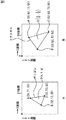••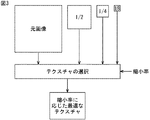•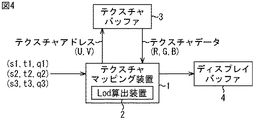•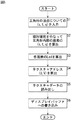••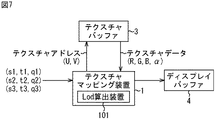•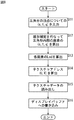•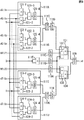•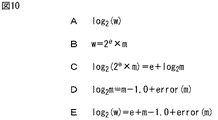•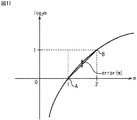••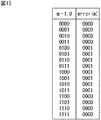•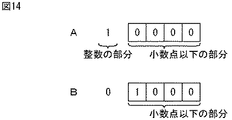•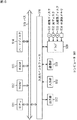## Classifications

• GPHYSICS
• G06COMPUTING; CALCULATING; COUNTING
• G06TIMAGE DATA PROCESSING OR GENERATION, IN GENERAL
• G06T15/003D [Three Dimensional] image rendering
• G06T15/04Texture mapping
• GPHYSICS
• G06COMPUTING; CALCULATING; COUNTING
• G06TIMAGE DATA PROCESSING OR GENERATION, IN GENERAL
• G06T2210/00Indexing scheme for image generation or computer graphics
• G06T2210/36Level of detail

## Description


BACKGROUND OF THE INVENTION
The present invention relates to an image processing apparatus and method, a recording medium, and a program, and more particularly, to an image processing apparatus and method, a recording medium, and a program that can calculate lod with a simple configuration apparatus.

[Prior art]
In computer graphics, when drawing a three-dimensional figure (model), a two-dimensional image (hereinafter referred to as texture) prepared in advance is pasted on the surface of the model to generate a high-quality image. There is a technique called texture mapping that can be done.

Here, the basic principle of texture mapping will be outlined with reference to FIGS.

The XY coordinates in FIG. 1A are coordinates to which the model to which the texture is attached is mapped.

The model to be drawn is formed by a set of triangular polygons as shown in FIG. 1A. Three-dimensional coordinate values (Sn, Tn, Qn, where n = 1, 2, 3) are given to the vertices A, B, and C of each polygon forming the model. In the following, although not shown, the three-dimensional coordinates are referred to as texture coordinates.

The coordinate value (s, t, q) of point D inside the polygon is obtained by linearly interpolating the texture coordinate values of points A, B, and C. The coordinates (s, t) are the homogeneous coordinates (s, t) of the texture indicating the image pattern of pasting, and the homogeneous term q is simply like an enlargement / reduction ratio.

The texture coordinate values such as (s, t, q) and (Sn, Tn, Qn) described above are given to the individual polygons constituting the model to be drawn and are variables. In addition, the vertex coordinate values (Sn, Tn, Qn, where n = 1, 2, 3) of the texture coordinate polygon correspond to the XY coordinate values (X, Y), and the texture coordinate value ( s, t, q) corresponds to XY coordinate values (x, y).

The UV coordinates in FIG. 1B are the two-dimensional coordinates of the texture pasted on the polygon of the model to be drawn. The UV coordinate values (u, v) of the texture points A ′, B ′, C ′, D ′ in FIG. 1B are multiplied by the homogeneous coordinates Q of the polygons (Sn, Tn) (Sn / Qn, Tn / Qn). 1B is pasted on the polygon so that the points A ′, B ′, C ′, D ′ correspond to the points A, B, C, D of the polygon mapped to the XY coordinates.

FIG. 2B shows how the side element dx in the X-axis direction (FIG. 2A) of the unit pixel constituting the XY coordinate corresponds to the UV coordinate, and the side element dx of the XY coordinate indicates the UV coordinate. This corresponds to a displacement amount of du / dx in the U-axis direction and dv / dx in the V-axis direction. That is, du / dx and dv / dx are the amount of displacement of u and the amount of change of v in the UV coordinates when (dx) changes in the XY coordinates. The same applies to the displacement on the UV coordinate corresponding to the side element dy (not shown) in the Y-axis direction.

Incidentally, MIPMAP filtering (Multum in parvo mapping) is known as a technique for obtaining high image quality when performing this texture mapping. MIPMAP filtering is described in detail in Advanced Animation and Rendering Techniques (page 140) Publisher: ADDISON WESLEY.

As shown in FIG. 3, the MIPMAP filtering includes a plurality of filter processes corresponding to each of a plurality of different reduction ratios (1/1, 1/2, 1/4, 1/8 in the example of FIG. 3). Prepared texture data (original image, 1/2 image, 1/4 image, 1/8 image) is prepared in advance, and optimum texture data corresponding to the reduction ratio of each pixel is selected and used. That is, it is possible to suppress the influence of aliasing due to information loss accompanying image reduction, and to obtain high image quality.

FIG. 4 shows a usage example of the texture mapping apparatus 1 that performs MIPMAP filtering.

The texture buffer 3 stores texture data subjected to a plurality of filter processes corresponding to a plurality of different reduction ratios as shown in FIG.

The texture mapping apparatus 1 calculates lod (Level Of Detail) representing the reduction rate of the polygon pixels. The texture mapping apparatus 1 reads an image corresponding to the calculated lod from the texture buffer 3, outputs it to the display buffer 4 and stores it. An image based on the recorded contents of the display buffer 4 is displayed on a display unit (not shown).

The operation of the texture mapping apparatus 1 will be described with reference to the flowchart of FIG.

In step S1, the texture mapping apparatus 1 shows the homogeneous coordinates and the homogeneous terms for each vertex of the polygon (FIG. 1A) (s1 , T1 , Q1 ), (S2 , T2 , Q2), And (sThree , TThree , QThree ).

Next, in step S2, the texture mapping apparatus 1 determines (s1 , T1 . q1 ), (S2 , T2 , Q2 ), And (sThree , TThree , QThree ) Is linearly interpolated to obtain (s, t, q) indicating the homogeneous coordinates and homogeneous terms of each pixel inside the polygon.

In step S <b> 3, the texture mapping apparatus 1 calculates the lod of each pixel from (s, t, q) of each pixel inside the polygon in the built-in lod calculation apparatus 2.

Here, as shown in Expression (1), it can be expressed by the logarithm of n when the reduction rate of (s, t, q) of each pixel is 1 / n with 2 as the base. Therefore, when the reduction ratio is 1/1, 1/2, 1/4, 1/8..., 0, 1, 2, 3,.
[Expression 1]

N of the reduction ratio (1 / n) can be obtained by Expression (2).
[Expression 2]

In the equation (2), du / dx and dv / dx are the amount of displacement of u and the amount of change of v in the UV coordinates when (dx) changes in the XY coordinates (FIG. 2B), and du / dy and dv / dy are the amount of displacement of u and the amount of change of v in the UV coordinates when (dy) changes in the XY coordinates, and are calculated according to Equation (3). Therefore, n can be obtained by Expression (4).
[Equation 3]
[Expression 4]

In equations (3) and (4), dS / dx, dT / dx, and dQ / dx are the differences in (S, T, Q) per pixel in the X direction, dS / dy, dT / dy, And dQ / dy represent the difference of (S, T, Q) per pixel in the Y direction. USIZE represents the width of the texture (length in the U direction), and VSIZE represents the height of the texture (length in the V direction).

Therefore, lod is finally calculated by calculating Expression (5) in which Expression (4) is substituted into Expression (1).
[Equation 5]

FIG. 6 illustrates a configuration example of the lod calculation device 2 that calculates the lod by calculating the equation (5).

The divider 11 divides 1 by the input Q (calculates 1 / Q), and the division result is multiplied by a multiplier 12, a multiplier 13, a circuit 21 (multipliers 32 and 34), and a circuit 22 ( Multipliers 42 and 44), circuit 24 (multipliers 52 and 54), and circuit 25 (multipliers 62 and 64).

The multiplier 12 multiplies S and 1 / Q, and outputs the multiplication result to the circuit 21 (multiplier 35) and the circuit 22 (multiplier 45). The multiplier 13 multiplies T by 1 / Q and outputs the multiplication result to the circuit 24 (multiplier 55) and the circuit 25 (multiplier 65).

The circuit 21 including the multiplier 31 to the absolute value detector 37 calculates a portion corresponding to the sign A in the equation (5) (hereinafter referred to as a portion A. The same applies to other portions), and the multiplier 41 Thru | or the absolute value detector 47 calculates the part C of Formula (5), and outputs a calculation result to the maximum value detector 23, respectively. The maximum value detector 23 detects the larger one of the value from the circuit 21 (value of the part A) and the value from the circuit 22 (value of the part C), and outputs the detection result to the maximum value detector 27. To do.

The circuit 24 composed of the multiplier 51 to the absolute value detector 57 computes the part B of the equation (5), and the circuit 25 composed of the multiplier 61 to the absolute value detector 67 computes the part D and obtains the computation result. Are output to the maximum value detector 26, respectively. The maximum value detector 26 detects the larger one of the value from the circuit 24 (part B value) and the value from the circuit 25 (part D value), and outputs the detection result to the maximum value detector 27. To do.

The maximum value detector 27 detects the larger one of the value from the maximum value detector 23 and the value from the maximum value detector 26, and outputs the detection result to the logarithmic calculator 28. The logarithmic calculator 28 calculates the logarithm of the value from the maximum value detector 27 with 2 as the base, and the calculation result (the calculation result of the entire expression (5)) is stored in the display buffer 4 (FIG. 4) as lod. Output.

Next, in step S4, the texture mapping apparatus 1 calculates u data obtained by dividing s data by q data and v data obtained by dividing t data by q data for (s, t, q) of each pixel. Then, texture coordinate data (u, v) is calculated.

In step S5, the texture mapping apparatus 1 obtains a texture address (U, V) which is a physical address in the texture buffer 3 from the lod calculated by the lod calculation apparatus 2 and the texture coordinate data (u, v), Output to buffer 3 to read texture data (R, G, B).

Next, in step S <b> 6, the texture mapping apparatus 1 writes pixel data obtained by performing predetermined processing on the texture data read in step S <b> 5 in the display buffer 4.

Thereafter, the process ends.

As described above, the access to the texture data corresponding to the lod among the plurality of texture data corresponding to each of the plurality of different reduction ratios stored in the texture buffer 3 is realized.

[Problems to be solved by the invention]
By the way, the part A to the part D of the formula (5) include Q2Is divided. As a result, the configuration of the lod calculation device 2 requires many multipliers in addition to the divider (one divider 11 in the example of FIG. 6) as shown in FIG. 6 (FIG. 6). In the case of the example, 22 multipliers are required), and there is a problem that the size of the lod calculation device 2 is increased.

The present invention has been made in view of such a situation, and makes it possible to calculate lod with an apparatus having a simple configuration.

[Means for Solving the Problems]
The image processing apparatus according to the present invention determines a storage unit that stores a plurality of texture data corresponding to a predetermined reduction ratio, and a reduction ratio of a pixel of a unit graphic based on a calculation result of a predetermined expression that does not include division. A determination unit, an acquisition unit for acquiring texture data corresponding to the reduction ratio determined by the determination unit from the storage unit, and an association unit for associating the texture data acquired by the acquisition unit with a unit graphic;The determination means is a floating-point value 2 of the value W, which is a logarithmic operation of an arbitrary value W with 2 as the base and 2 as the base. e It is performed by calculating the logarithm of xm.

The determination means is 2 with 2 as the base when 1 <m <2.eThe logarithm of xm, e, (m−1), and log2It can be calculated based on the difference value between m and m-1.

The determining means stores a table made up of m and a difference value corresponding to m, with 2 being the base 2eThe logarithm of xm can be calculated based on e, (m−1), and the difference value corresponding to m in the table.

According to the image processing method of the present invention, a storage step for storing a plurality of texture data corresponding to a predetermined reduction ratio and a reduction ratio of a pixel of a unit graphic are determined based on a calculation result of a predetermined expression not including division. A determination step, an acquisition step of acquiring texture data corresponding to the reduction ratio determined in the processing of the determination step from a plurality of texture data stored in the processing of the storage control step, and a texture acquired in the processing of the acquisition step An association step of associating data with the unit figure,The decision step is a logarithmic operation of an arbitrary value W with 2 as a base included in the formula, and is a floating-point value 2 of the value W with 2 as a base. e It is performed by calculating the logarithm of xm.

The recording medium program according to the present invention includes a storage control step for controlling storage of a plurality of texture data corresponding to a predetermined reduction ratio, and a calculation result of a predetermined expression that does not include division of the pixel figure reduction ratio. A determination control step for controlling the determination based on, and an acquisition control step for controlling the acquisition of the texture data corresponding to the reduction ratio determined in the processing of the determination control step from the plurality of texture data stored in the processing of the storage control step And an association control step for controlling the association of the texture data acquired in the acquisition control step with the unit graphic,The decision control step is a logarithmic operation of an arbitrary value W, with 2 as a base included in the expression, and is a floating-point value 2 of the value W with 2 as a base. e It is performed by calculating the logarithm of xm.

The program of the present invention determines a storage control step for controlling storage of a plurality of texture data corresponding to a predetermined reduction ratio, and determines a reduction ratio of a pixel of a unit graphic based on a calculation result of a predetermined expression not including division. A determination control step for controlling, an acquisition control step for controlling acquisition of the texture data corresponding to the reduction ratio determined in the processing of the determination control step from a plurality of texture data stored in the processing of the storage control step, and acquisition An association control step for controlling the association of the texture data acquired in the process of the control step with the unit graphic,The decision control step is a logarithmic operation of an arbitrary value W, with 2 as a base included in the expression, and is a floating-point value 2 of the value W with 2 as a base. e It is characterized by causing a computer to execute processing performed by calculating a logarithm of xm.

In the image processing apparatus, method, and program of the present invention, a plurality of texture data corresponding to a predetermined reduction ratio is stored, and the reduction ratio of the pixel of the unit graphic is based on a calculation result of a predetermined expression not including division. Texture data corresponding to the determined reduction ratio is acquired from the plurality of stored texture data, the acquired texture data is associated with the unit graphic,Determination of the reduction ratio of the pixel of the unit graphic is a floating point value of the value W, where the logarithm operation of an arbitrary value W with 2 as the base included in the equation is 2 e This is done by calculating the logarithm of × m.

DETAILED DESCRIPTION OF THE INVENTION
FIG. 7 shows an example of use of the texture mapping apparatus 1 to which the present invention is applied. The texture mapping apparatus 1 is provided with a lod calculation apparatus 101 in place of the lod calculation apparatus 2 of FIG.

Next, the operation of the texture mapping apparatus 1 will be described with reference to the flowchart of FIG.

In steps S11 and S12 and steps S14 to S16, the same processes as those in steps S1 and S2 and steps S4 to S6 of FIG.

In step S <b> 13, the lod calculation apparatus 101 calculates lod by calculating Expression (6) from (s, t, q) of each pixel inside the polygon.
[Formula 6]

Expression (6) is an expression (5) calculated to calculate lod in the lod calculation device 2 of FIG. 4 developed so as not to include division. To be exact, expression (5) Is expanded as shown in Expression (7), and further expanded as shown in Expression (6).
[Expression 7]

FIG. 9 illustrates a configuration example of the lod calculation apparatus 101. The lod calculation device 101 has 22 multipliers compared to the lod calculation device 2 of FIG. 4, but only nine of them are provided, so that the size of the device is reduced.

Each of the circuits 111A to 111C in FIG. 9 includes two multipliers, one subtractor, and one absolute value detector.

The circuit 111A composed of the multiplier 121-1 to the absolute value detector 121-4 calculates the part A of the equation (6) and outputs the calculation result to the maximum value detector 111C. That is, the multiplier 121-1 multiplies dS / dx and Q, the multiplier 121-2 multiplies dQ / dx and S, and outputs the multiplication result to the subtractor 121-3. The subtractor 121-3 subtracts the multiplication result from the multiplier 121-2 from the multiplication result from the multiplier 121-1, and outputs the subtraction result to the absolute value detector 121-4. The absolute value detector 121-4 detects the absolute value of the subtraction result from the subtractor 121-3, and outputs the detection result to the maximum value detector 111C.

A circuit 111B composed of the multiplier 122-1 to the absolute value detector 122-4 calculates the part B of Expression (6) and outputs the calculation result to the maximum value detector 111C.

The maximum value detector 111C detects the larger of the calculation result from the circuit 111A (the value of the part A in the expression (6)) and the calculation result from the circuit 111B (the value of the part B in the expression (6)). Output to the logarithmic calculator 111D. That is, the maximum value detector 111C calculates the part C of the formula (6).

The logarithmic calculator 111D calculates the logarithm of the value from the maximum value detector 111C with 2 as the base (calculates the part D of the equation (6)), and outputs the calculation result to the adder 131.

The logarithmic calculator 111E calculates the logarithm of USIZE with 2 as the base (calculates the part E of the equation (6)), and outputs the calculation result to the subtractor 132.

Multiplier 111F calculates the square of Q (calculates part F of equation (6)), and outputs the calculation result to logarithmic calculator 111G. The logarithmic calculator 111G calculates the logarithm of the square of Q with 2 as the base (calculates the part G), and outputs the calculation result to the subtracter 132 and the subtracter 133.

The logarithmic calculator 111H calculates the logarithm of VSIZE with 2 as the base (calculates the part H of the equation (6)), and outputs the calculation result to the subtracter 133.

The circuit 111I composed of the multiplier 123-1 to the absolute value detector 123-4 calculates the part I of the equation (6), and the circuit 111J composed of the multiplier 124-1 to the absolute value detector 124-4 J is calculated, and the calculation result is output to the maximum value detector 111K.

The maximum value detector 111K detects the larger one of the operation result from the circuit 111I (the value of the part I in the expression (6)) and the operation result from the circuit 111J (the value of the part J in the expression (6)). (Part K is calculated) and output to the logarithmic calculator 111L. The logarithm calculator 111L calculates the logarithm of the value from the maximum value detector 111K with 2 as the base (calculates the part L), and outputs the calculation result to the adder 134.

The subtractor 132 subtracts the value from the logarithmic calculator 111G from the value from the logarithmic calculator 111E, and outputs the subtraction result to the adder 131. The adder 131 adds the value from the logarithmic calculator 111D and the value from the subtractor 132, and outputs the addition result to the maximum value detector 135.

The subtracter 133 subtracts the value from the logarithmic calculator 111G from the value from the logarithmic calculator 111H, and outputs the subtraction result to the adder 134.

The adder 134 adds the value from the logarithmic calculator 111 </ b> L and the value from the subtracter 133, and outputs the addition result to the maximum value detector 135.

The maximum value detector 135 detects the larger one of the value from the adder 131 and the value from the adder 134, and displays the detection result (the calculation result of the entire formula (6)) as lod as a display buffer. 4 is output.

By the way, the logarithmic calculators 111D, E, G, H, and L calculate the logarithm of the input value (hereinafter referred to as the value W) with 2 as the base, as shown in the equation of FIG. 10A. Since the input value to the lod calculation device 101 is a floating-point number, a logarithmic operation of a floating-point number is performed here.

In the following, the logarithmic operation of this floating point number will be described.

The floating point value of the value W is expressed as shown in FIG. 10B, and the right side of FIG. 10C is obtained by taking the logarithm with 2 as the base, as in the left side of FIG. 10C.

By the way, the second term on the right side of FIG.2The trajectory of m) is as shown in FIG. That is, the second term on the right side of FIG. 10C can be approximated to a straight line passing through point A and point B in FIG. 11 within the range of 1 <m <2, and therefore the equation of FIG. 10D is established.

Error (m) in the equation of FIG. 10D is the difference between the second term on the right side of FIG. 10C and the straight line passing through points A and B in FIG.2m is the difference between (m−1.0)).

That is, when 1 <m <2, the logarithm calculator obtains e and m that constitute the floating-point value of the value W, and error (m) corresponding to m, and FIG. 10D is displayed on the right side of FIG. 10C. By substituting (after all, the right side of FIG. 10E is calculated), the logarithmic value of FIG. 10A can be obtained.

Note that error (m) (the equations in FIGS. 10D and 10E, FIG. 11) corresponds to m on a one-to-one basis. Therefore, in this example, the logarithmic calculator has one m and error (m). Is stored in a correspondence table (details will be described later, to be precise, a correspondence table in which m-1 and error (m) are associated with one body 1). It is assumed that error (m) corresponding to m is acquired from the correspondence table.

In addition, in the addition of e, (m−1.0), and error (m) (the calculation on the right side of FIG. 10E), e is an integer, and (m−1.0) + error (m) is 1 Since the value is less than the decimal point under <m <2, in this example, as shown in FIG. 12, e is represented by 4 bits and set to the integer part, and (m−1.0 + error (m) ) Is represented by 4 bits and set to the decimal part. That is, lod consists of a 4-bit integer part and a 4-bit decimal part.

The addition of (m-1.0) and error (m) is performed by adding 4 bits representing m-1.0 and 4 bits representing error (m). That is, in this example, as shown in FIG. 13, the correspondence table includes 4 bits representing (m−1.0) as a numerical value after the decimal point and error (m) as a numerical value corresponding to the decimal point. The corresponding 4 bits are set. As m-1.0 is 0 or 1, that is, m is a value closer to 1 or 2, error (m) is smaller as shown in FIG.

For example, when m-1.0 is a value of 0.5 (when m = 1.5), since the 4 bits representing m-1.0 are “1000”, “1000” is represented by m in the correspondence table. “0001” in the column of error (m) set corresponding to “1000” in the column of −1.0 is added, and “1001” which is the addition result becomes a decimal part.

When the value 1 is represented by 1 bit representing an integer and 4 bits representing a numerical value after the decimal point as shown in FIG. 14A, the value 0.5 is the integer of FIG. 14A as shown in FIG. 14B. Since 1 set to 1 bit to be represented is shifted by 1 bit in the right direction in the figure (because 1 is halved), the value 0.5 is “1000”.

The series of processes described above can be realized by hardware, but can also be realized by software. When the series of processing is realized by software, a program constituting the software is installed in a computer, and the program is executed by the computer, so that the above-described lod calculation apparatus 101 is functionally realized. .

FIG. 15 is a block diagram illustrating a configuration of an embodiment of a computer 501 that functions as the lod calculation apparatus 101 as described above. An input / output interface 516 is connected to a CPU (Central Processing Unit) 511 via a bus 515, and the CPU 511 receives a command from an input unit 518 such as a keyboard and a mouse via the input / output interface 516. When input, it is stored in a recording medium such as a read only memory (ROM) 512, a hard disk 514, or a magnetic disk 531, an optical disk 532, a magneto-optical disk 533, or a semiconductor memory 534 mounted in the drive 520. The program is loaded into a RAM (Random Access Memory) 513 and executed. Thereby, the various processes described above are performed. Further, the CPU 511 outputs the processing result to an output unit 517 such as an LCD (Liquid Crystal Display) via the input / output interface 516 as necessary. The program is stored in advance in the hard disk 514 or ROM 512 and provided to the user integrally with the computer 501 or as a package medium such as the magnetic disk 531, the optical disk 532, the magneto-optical disk 533, and the semiconductor memory 534. Or can be provided to the hard disk 514 via the communication unit 519 from a satellite, a network, or the like.

In the present specification, the step of describing the program provided by the recording medium is not limited to the processing performed in chronological order according to the described order, but is not necessarily performed in chronological order. It also includes processes that are executed individually.

Further, in this specification, the system represents the entire apparatus constituted by a plurality of apparatuses.

【The invention's effect】
According to the present invention,The apparatus can have a simple configuration.
[Brief description of the drawings]
FIG. 1 is a diagram for explaining the principle of texture mapping.
FIG. 2 is another diagram illustrating the principle of texture mapping.
FIG. 3 is a diagram illustrating the principle of MIPMAP filtering.
FIG. 4 is a block diagram illustrating a configuration example of a conventional texture mapping apparatus.
FIG. 5 is a flowchart for explaining the operation of the texture mapping apparatus of FIG. 4;
6 is a block diagram illustrating a configuration example of a lod calculation device 2 in FIG. 4;
FIG. 7 is a block diagram illustrating a configuration example of a texture mapping apparatus to which the present invention is applied.
FIG. 8 is a flowchart for explaining the operation of the texture mapping apparatus in FIG. 7;
9 is a block diagram illustrating a configuration example of the lod calculation device in FIG. 7;
10 is a diagram for explaining a lod calculation method in the lod calculation apparatus of FIG. 7;
FIG. 11 log2It is a figure which shows the relationship between m and m.
FIG. 12 is a diagram illustrating a data configuration of lod.
FIG. 13 is a diagram for explaining a correspondence table;
FIG. 14 is a diagram illustrating a decimal part.
15 is a block diagram illustrating a configuration example of a personal computer 501. FIG.
[Explanation of symbols]
1 texture mapping device, 3 texture buffer, 4 display buffer, 101 lod calculation device, 111A circuit, 111B circuit, 111C maximum value detector, 111D logarithmic calculator, 111E logarithmic calculator, 111F multiplier, 111G logarithmic calculator, 111H Logarithmic calculator, 111I circuit, 111J circuit, 111K maximum value detector, 111L logarithmic calculator, 131 adder, 132 subtractor, 133 subtractor, 134 adder, 135 maximum value detector

## Claims (6)

1. In an image processing apparatus for determining a reduction rate for each pixel of a plurality of unit graphics forming a model, and associating texture data corresponding to the determined reduction rate with the unit graphic,
Storage means for storing a plurality of texture data corresponding to a predetermined reduction ratio;
Determining means for determining a reduction rate of the pixel of the unit graphic based on a calculation result of a predetermined expression not including division;
Obtaining means for obtaining the texture data corresponding to the reduction ratio determined by the determining means from the storage means;
Association means for associating the texture data acquired by the acquisition means with the unit graphic;
The determining means calculates a logarithm of an arbitrary value W with 2 as a base included in the formula, and calculates a logarithm of 2 e × m that is a floating point value of the value W with a base of 2. An image processing apparatus characterized in that the processing is performed .
2. The determining means calculates the logarithm of 2 e × m with 2 as the base when 1 <m <2, based on e, (m−1), and a difference value between log 2 m and m−1. The image processing apparatus according to claim 1.
3. The determining means stores a table including m and the difference value corresponding to m, and the logarithm of 2 e × m with 2 as a base is expressed as e, the (m−1), and the The image processing apparatus according to claim 1, wherein calculation is performed based on the difference value corresponding to the m in the table.
4. In the image processing method of the image processing apparatus for determining a reduction rate for each pixel of a plurality of unit graphics forming a model, and associating texture data corresponding to the determined reduction rate with the unit graphic,
A storage step of storing a plurality of texture data corresponding to a predetermined reduction ratio;
A determination step of determining a reduction rate of the pixel of the unit graphic based on a calculation result of a predetermined expression not including division;
An acquisition step of acquiring the texture data corresponding to the reduction ratio determined in the processing of the determination step from the plurality of texture data stored in the processing of the storage control step;
Associating the texture data acquired in the processing of the acquisition step with the unit graphic; and
The determination step calculates a logarithm of an arbitrary value W with 2 as a base included in the formula, and calculates a logarithm of 2 e × m that is a floating point value of the value W with 2 as a base. An image processing method characterized by being performed .
5. A program for an image processing apparatus that determines a reduction rate for each pixel of a plurality of unit graphics forming a model, and associates texture data corresponding to the determined reduction rate with the unit graphic,
A storage control step for controlling storage of a plurality of texture data corresponding to a predetermined reduction ratio;
A determination control step for controlling determination of a reduction ratio of the pixel of the unit graphic based on a calculation result of a predetermined expression not including division;
An acquisition control step for controlling acquisition of the texture data corresponding to the reduction ratio determined in the determination control step processing from the plurality of texture data stored in the storage control step processing;
An association control step for controlling the association of the texture data acquired in the acquisition control step with the unit graphic;
The determination control step calculates a logarithm of an arbitrary value W with 2 as a base included in the formula, and calculates a logarithm of 2 e × m that is a floating point value of the value W with 2 as a base. recording medium from which a computer readable program which is characterized in that by calculating is recorded.
6. A program for an image processing apparatus that determines a reduction rate for each pixel of a plurality of unit graphics forming a model, and associates texture data corresponding to the determined reduction rate with the unit graphic,
A storage control step for controlling storage of a plurality of texture data corresponding to a predetermined reduction ratio;
A determination control step for controlling determination of a reduction ratio of the pixel of the unit graphic based on a calculation result of a predetermined expression not including division;
An acquisition control step for controlling acquisition of the texture data corresponding to the reduction ratio determined in the determination control step processing from the plurality of texture data stored in the storage control step processing;
An association control step for controlling the association of the texture data acquired in the process of the acquisition control step with the unit graphic,
The determination control step calculates a logarithm of an arbitrary value W with 2 as a base included in the formula, and calculates a logarithm of 2 e × m that is a floating point value of the value W with 2 as a base. A program characterized by causing a computer to execute processing performed by calculation .
JP2001355866A 2001-11-21 2001-11-21 Image processing apparatus and method, recording medium, and program Expired - Fee Related JP3775580B2 (en)

## Priority Applications (1)

Application Number Priority Date Filing Date Title
JP2001355866A JP3775580B2 (en) 2001-11-21 2001-11-21 Image processing apparatus and method, recording medium, and program

## Applications Claiming Priority (2)

Application Number Priority Date Filing Date Title
JP2001355866A JP3775580B2 (en) 2001-11-21 2001-11-21 Image processing apparatus and method, recording medium, and program
US10/299,297 US7151862B2 (en) 2001-11-21 2002-11-19 Image processing apparatus and method, storage medium, and program

## Publications (2)

Publication Number Publication Date
JP2003157445A JP2003157445A (en) 2003-05-30
JP3775580B2 true JP3775580B2 (en) 2006-05-17

# Family

## Family Applications (1)

Application Number Title Priority Date Filing Date
JP2001355866A Expired - Fee Related JP3775580B2 (en) 2001-11-21 2001-11-21 Image processing apparatus and method, recording medium, and program

## Country Status (2)

US (1) US7151862B2 (en)
JP (1) JP3775580B2 (en)

## Families Citing this family (4)

* Cited by examiner, † Cited by third party
Publication number Priority date Publication date Assignee Title
JP4140575B2 (en) * 2004-07-30 2008-08-27 ソニー株式会社 Image deformation device, image deformation circuit, and image deformation method
JP4462132B2 (en) 2005-07-04 2010-05-12 ソニー株式会社 Image special effects device, graphics processor, program
US8207980B2 (en) * 2007-05-01 2012-06-26 Vivante Corporation Coordinate computations for non-power of 2 texture maps
US8106918B2 (en) * 2007-05-01 2012-01-31 Vivante Corporation Apparatus and method for texture level of detail computation

## Family Cites Families (8)

* Cited by examiner, † Cited by third party
Publication number Priority date Publication date Assignee Title
JP3082289B2 (en) * 1991-05-14 2000-08-28 富士ゼロックス株式会社 Image processing device
JP2637931B2 (en) * 1994-12-01 1997-08-06 インターナショナル・ビジネス・マシーンズ・コーポレイション Computer system for texture mapping
KR100214332B1 (en) * 1996-09-02 1999-08-02 윤종용 Method for changing image ratio
JP4069486B2 (en) * 1998-03-17 2008-04-02 ソニー株式会社 Memory circuit control device and graphic operation device
JP4300650B2 (en) * 1999-09-07 2009-07-22 ソニー株式会社 Arithmetic processing circuit and method and image processing apparatus
JP3502024B2 (en) * 1999-09-10 2004-03-02 株式会社ソニー・コンピュータエンタテインメント Image processing apparatus, recording medium, and image processing method
JP3352982B2 (en) * 1999-09-14 2002-12-03 株式会社スクウェア Rendering method and device, game device, and computer-readable recording medium for storing program for rendering three-dimensional model
JP4568950B2 (en) * 2000-02-29 2010-10-27 ソニー株式会社 Graphics drawing device

## Also Published As

Publication number Publication date
US7151862B2 (en) 2006-12-19
US20030117399A1 (en) 2003-06-26
JP2003157445A (en) 2003-05-30

## Similar Documents

Publication Publication Date Title
US9652882B2 (en) Gradient adjustment for texture mapping for multiple render targets with resolution that varies by screen location
US10269176B2 (en) Efficient geometric tessellation and displacement
EP2381421B1 (en) Automatic generation of 3D models from packaged goods product images
US7050068B1 (en) Generation of jittered sub-pixel samples using programmable sub-pixel offsets
US5808619A (en) Real-time rendering method of selectively performing bump mapping and phong shading processes and apparatus therefor
US10685420B2 (en) Tile based computer graphics
US6078331A (en) Method and system for efficiently drawing subdivision surfaces for 3D graphics
DE60126967T2 (en) Method and apparatus for over-sampling anti-aliasing
JP3294149B2 (en) Three-dimensional texture mapping processing device and three-dimensional image generation device using the same
US5278949A (en) Polygon renderer which determines the coordinates of polygon edges to sub-pixel resolution in the X,Y and Z coordinates directions
US6246422B1 (en) Efficient method for storing texture maps in multi-bank memory
US6999095B2 (en) System and process for optimal texture map reconstruction from multiple views
US6141013A (en) Rapid computation of local eye vectors in a fixed point lighting unit
Park et al. A method for approximate NURBS curve compatibility based on multiple curve refitting
US5343558A (en) Method for scan converting shaded triangular polygons
US7081895B2 (en) Systems and methods of multi-pass data processing
TW201601115A (en) Varying effective resolution by screen location in graphics processing by approximating projection of vertices onto curved viewport
JP3245655B2 (en) Workspace display processing method
US6133901A (en) Method and system for width independent antialiasing
JP4205327B2 (en) Volume dataset rendering method and system
US6160557A (en) Method and apparatus providing efficient rasterization with data dependent adaptations
US10140762B2 (en) Tessellation method
KR101033779B1 (en) Method and apparatus for processing images using graphics processing unit with extended vertex cache
US5905500A (en) Method and apparatus for adaptive nonlinear projective rendering
US7280106B2 (en) Apparatus and method for generating texture maps for use in 3D computer graphics

## Legal Events

Date Code Title Description
A977 Report on retrieval

Free format text: JAPANESE INTERMEDIATE CODE: A971007

Effective date: 20051021

A131 Notification of reasons for refusal

Free format text: JAPANESE INTERMEDIATE CODE: A131

Effective date: 20051028

A521 Written amendment

Free format text: JAPANESE INTERMEDIATE CODE: A523

Effective date: 20051227

TRDD Decision of grant or rejection written
A01 Written decision to grant a patent or to grant a registration (utility model)

Free format text: JAPANESE INTERMEDIATE CODE: A01

Effective date: 20060202

A61 First payment of annual fees (during grant procedure)

Free format text: JAPANESE INTERMEDIATE CODE: A61

Effective date: 20060215

LAPS Cancellation because of no payment of annual fees